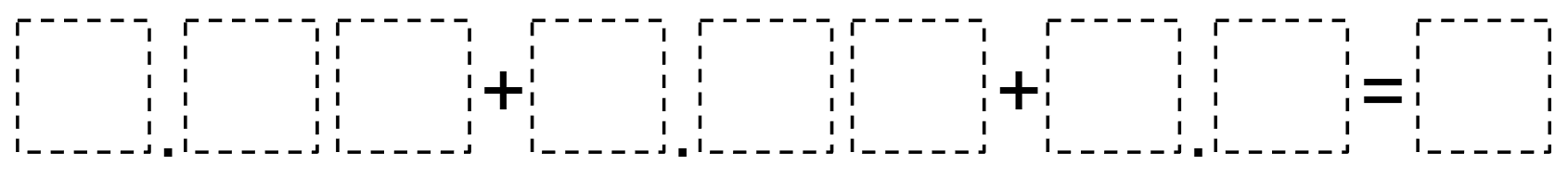# Adding Parts of a Whole

Directions: Using the digits 1 to 9, at most one time each, make the following statement true.### Hint

How can you make the hundredths a tenth?

There are many solutions that sum to 9 4.87+2.63+1.5=9, 2.83 + 4.57 + 1.6 = 9.

Source: Miles Knight

## Multiplying Two-Digit Numbers – Closest to 7,000

Directions: Directions: Using the digits 1 to 9 at most one time each, fill in …

1.1.41+1.09+1.5=4

•You can only use each digit once though, Alejandro.

2.1,5+2,7+3,8=8

3.4.5.•6.